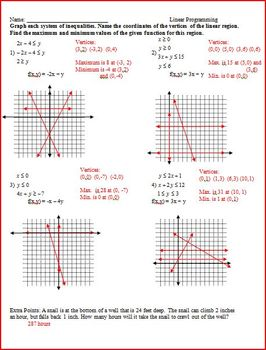Parent Graphs (Families of Graphs) and Linear Programming (WS)Subject
Resource Type
Product Rating
4.0
2 Ratings
File Type

Word Document File

264 KB|12 pages
Share
Product Description
This is a set of 4 worksheets.

1 worksheet is good for an introduction to families of graphs (or parent graphs). There are 9 questions asking to identify the parent graph and describe the transformations. There is also 11 review questions (writing equations, finding the intercepts, and plugging in values).

1 worksheet is graphing parent graphs. There are 6 questions to state the parent graph, describe the transformations, and graph the equations and the parent graph.

1 worksheet is a first day of using a function to determine the max and min value of feasible regions. There are 6 questions plugging in ordered pairs and 2 questions that are word problems and a max or min needs to be calculated.

1 worksheet is 4 questions for students to graph the equations to create the feasible region and then use the function and determine the max and min.

Answer key is included for all sheets.

Total Pages
12 pages